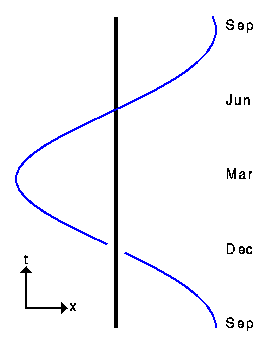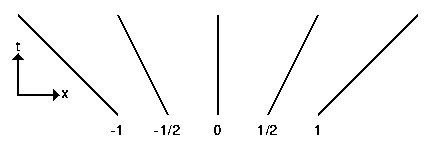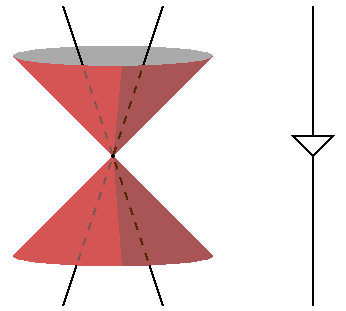# Space-Time Diagrams

Any discussion of cosmology requires a careful consideration of what we can see and when we can see it. A good way to keep track of these concepts is the space-time diagram. A space-time diagram is nothing more than a graph showing the position of objects as a function of time. The usual convention is that time runs up the diagram, so the bottom is the past, or early times, and the top is the future, or late times. A point on this graph describes both a position (the horizontal or x coordinate) and a time (the vertical or t coordinate). A "point" in space-time is called an event.The picture above shows a space-time diagram of the Earth going around the Sun. This figure uses perspective to try to show two spatial dimensions and the time axis on a two dimensional sheet of paper, but usually we will just show one spatial axis and avoid perspective.

The line representing the position of the Earth as a function of time is called a worldline. The slope of the worldline for a particle shows its velocity in the reference frame of the diagram.The space-time diagram above shows particles with different velocities.Because the speed of light is special in relativity, space-time diagrams are often drawn in units of seconds and light-seconds, or years and light-years, so a unit slope [45 degree angle] corresponds to the speed of light. The set of all light speed world lines going through an event defines the light cones of that event: the past light cone and the future light cone. An example of light cones is shown above. The fancy light picture on the left shows both the past and future light cones of the event where the two worldlines cross, while the schematic version on the right is easy to use in more complicated diagrams.

Back to the Cosmology Tutorial